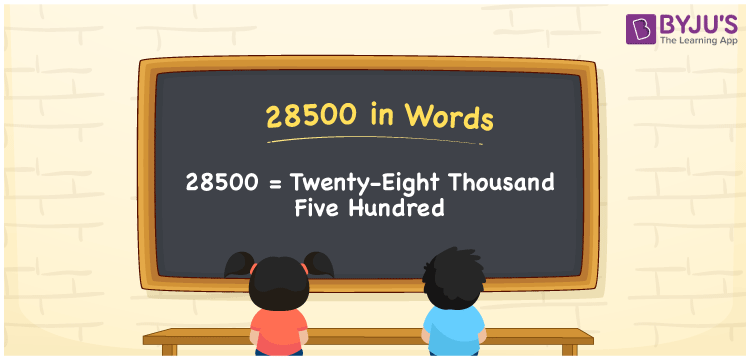# 28500 in Words

28500 in words can be written as Twenty-eight thousand five hundred. The article of 28500 in words will provide students with a clear knowledge of the concept of counting numbers. If Rs. 28500 is spent on buying new clothes, then you can say that “I spent Twenty-eight thousand five hundred Rupees in buying new clothes”. The  concept of converting 28500 into words using the English alphabet is discussed here. Students will be able to convert numbers in words with the help of the resources available at BYJU’S. 28500 can be read as “Twenty-eight thousand five hundred” in English.

 28500 in words Twenty-eight thousand five hundred Twenty-eight thousand five hundred in Numbers 28500

## 28500 in English Words## How to Write 28500 in Words?

In this section you will learn in detail about conversion of 28500 into words using the place value chart. Five digits are present in 28500. The place value chart of 28500 can be understood easily using the table below.

 Ten Thousands Thousands Hundreds Tens Ones 2 8 5 0 0

The expanded form of the number 28500 is given below:

2 x Ten Thousand + 8 × Thousand + 5 × Hundred + 0 × Ten + 0 × One

= 2 x 10000 + 8 × 1000  + 5 × 100 + 0 × 10 + 0 × 1

= 20000 + 8000 + 500

= 28500

= Twenty-eight thousand five hundred

Hence, 28500 in words is written as Twenty-eight thousand five hundred.

28500 is a natural number that precedes 28501 and succeeds 28499.

28500 in words – Twenty-eight thousand five hundred

Is 28500 an odd number? – No

Is 28500 an even number? – Yes

Is 28500 a perfect square number? – No

Is 28500 a perfect cube number? – No

Is 28500 a prime number? – No

Is 28500 a composite number? – Yes

## Frequently Asked Questions on 28500 in Words

Q1

### Write 28500 in words.

28500 can be written as “Twenty-eight thousand five Hundred” in words.
Q2

### Is 28500 an odd or even number?

28500 is an even number because it is divisible by 2.
28500/2 = 14250
Q3

### Write Twenty-eight thousand five Hundred in numbers.

Twenty-eight thousand five Hundred can be written in numbers as 28500.